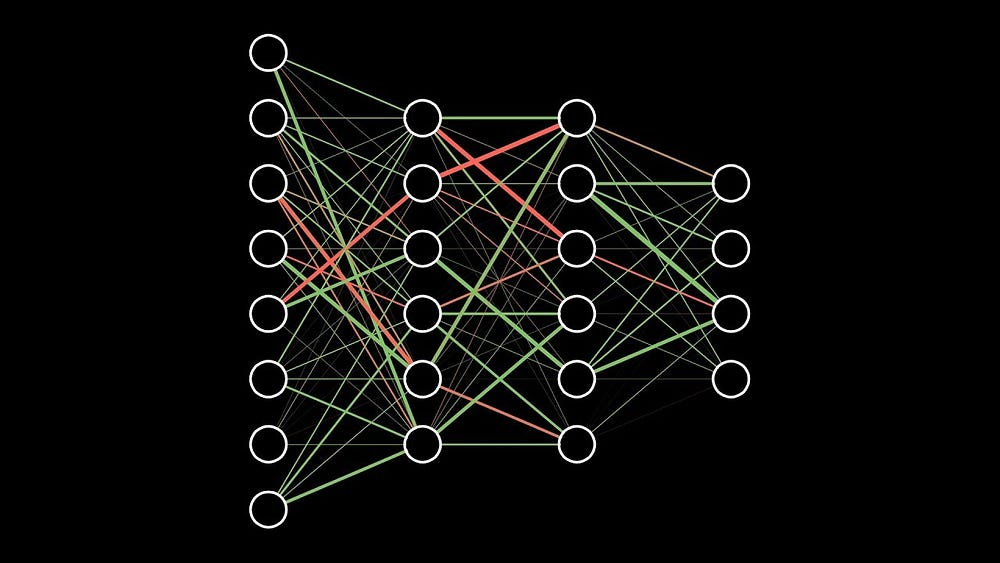# We might have to ditch backpropagation

## Introduction

An artificial neural network is characterized by the weights & biases of its layers. These weights and biases are responsible for mapping the given input to the respective output. Generally, they are randomly initialized to small numbers close to 0 (although there are several ways to optimally initialize the network’s weights & biases, we will not be focusing on it in this article), and are updated in a process which we call “training the network”, to minimize the error.

Backpropagation is a method used for updating these weights & biases to gain better accuracy. It works by calculating the gradient of the cost function with respect to the network parameters (weights and biases of different layers) & updating the values by a small amount in the opposite direction of the gradient i.e. in the direction which takes us to the local minima of the cost function.

History

The basics of continuous backpropagation were derived by Henry Kelly in 1960 and by Arthur Bryson in 1961 in the context of control theory. They used the principles of dynamic programming.

In 1962, Stuart Dreyfus published a simpler derivation based only on the chain rule, and in 1968, David Rumelhart, Geoffrey Hinton and Ronald Williams showed experimentally that this method can generate useful internal representations of incoming data in hidden layers of neural networks. Simply put, they showed that this method can be used to calculate the updates for the network parameters which lead to the convergence to the local minima of the cost function.

#### So, what’s wrong?

In a recent AI conference, Hinton remarked that he was “deeply suspicious” of back-propagation, and said “My view is throw it all away and start again.”

Backpropagation is great due to several reasons – elegant maths, differentiable objective function, easy to update model parameters, etc. However, there are a few problems with backpropagation:

Is the gradient that is calculated always in the correct direction towards learning? This is a very intuitive question — one can always find problems wherein moving towards the most obvious direction does not always lead to a solution. So it should not be surprising that ignoring the gradient may also lead to a solution.

Synthetic Gradients, an approach that decouples layers so that the gradient calculation can be delayed, has also shown to be equally effective. This finding may be a hint that something else more general is going on. It is as if any update that tends to be incremental, regardless of the direction (random in the case of synthetic gradients) works equally well.

There is another problem, regarding the objective function that is employed: Backpropagation is calculated with respect to some objective function. Usually, the objective function is a measure of the difference between the predicted output and the actual output. This means that the ground truth (actual output) must be known. This is the case in the supervised learning domain, however, the real world is not purely ‘supervised’ per se.

To sum up, backpropagation is not possible if you don’t have an objective function. You can’t have an objective function if you don’t have a measure between the predicted value and the actual value. So, to be able to achieve “unsupervised learning”, you may have to ditch the ability to calculate the gradient.

Unsupervised learning represents real problems with serious challenges, in that sense, maybe backpropagation is not enough and a change in paradigm is imminent to pave the way for the next breakthrough.